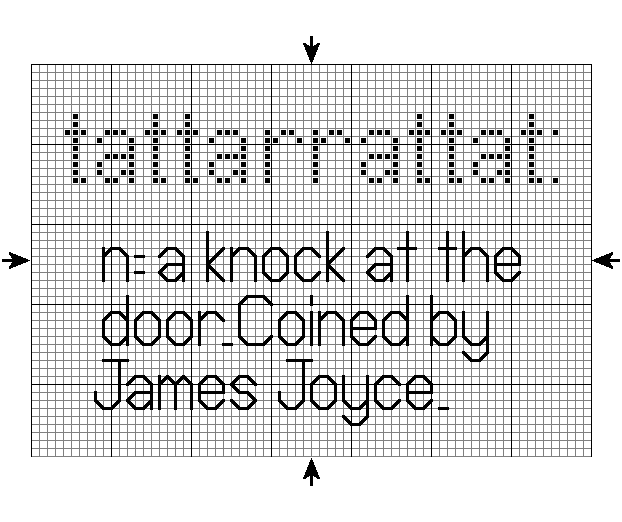# Palindrome Program In Java – 5 Ways | Programs

Palindrome program in Java – Here, we will discuss the various methods to check whether a given number is a palindrome or not. A compiler is added so that you can execute the program yourself, alongside various examples and sample outputs are given. The methods aforementioned are:

• Print using For Loop
• Print using Static Method
• Print using Recursion
• Print using String

A Palindrome is a sequence of words, digits or other symbols that reads the same both forwards and backwards.

There are several examples such as RACECAR, MOM, 12321 etc.

This is another example of a palindrome:The speciality of this palindrome is that this is the longest palindrome in the existence of the English Language. This palindrome was termed by James Joyce in Ulysses in 1922.

The meaning of this word is simply a knock at the door.

Thus, the methods with which the string is a palindrome or not be determined in Java programs are as follows:

## Using For Loop

1) The number is called a palindrome if a number is equal to reverse of its number.

2) For loop repeats rem=num%10;, s=(s*10)+rem these steps until num>0. If the condition is false, then it compares the s value with t, if both are equal then it prints the given number is a palindrome.

Output:

### Using Static Method

1) In this program we have the static method palindromeOrNot(int num ), it calculates the reverse of the given number.

2) Call the palindromeOrNot(int num ) method in the main method. In this method while loop repeats  r=num%10; sum=(sum*10)+r; num/=10; these  steps until num!=0 is false. If num=0 then it returns the sum, then compare the original number with this reverse number, if both are equal then it prints given number is a palindrome.

Output:

#### Using Recursion

1) Using the “Palin” object p, we will call the palindromeOrNot(a) method.

2) The method palindromeOrNot(int num) calls itself as palindromeOrNot(num) until num!=0, if num=0 then it returns sum and sum assigned to s and compares with t, if both are equal then it prints message as “palindrome number”.

Output:

##### Using String

1) Here we are comparing the two strings.

2) Entered string will be assigned to str variable, here str is string datatype.

3) For loop iterates until i>=0, here i=length of the string -1. length() is the string class method.

i=length-1=2 then  strrev=””+d =d (here charAt(2) i.e character at the index 2 is d)

i=1 then strrev=d+a(here charAt(1) i.e character at the index 1 is a)

i=0 then strrev=da+D(here charAt(0) i.e character at the index 0 is D)

4) After all iterations srtrev=daD, now compare the two strings using strrev.equalsIgnoreCase(str), this is string class method, it compares the characters available in strrev string with str. If both the characters are equal then prints entered string is palindrome.

Output:

More Java Programs:

x

## Java Program to Add Two Matrices – 4 Ways | Programs

Java program to add two matrices – The following Java Code will let you know ...